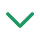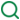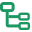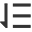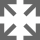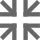## 一、迭代器

JavaScript 原有的表示“集合”的数据结构，主要是数组（Array）和对象（Object），ES6 又添加了Map和Set。这样就需要一种统一的接口机制，来处理所有不同的数据结构。遍历器（Iterator）就是这样一种机制。它是一种接口，为各种不同的数据结构提供统一的访问机制。任何数据结构只要部署 Iterator 接口，就可以完成遍历操作（即依次处理该数据结构的所有成员）

### 1.Iterator的作用：

• 为各种数据结构，提供一个统一的、简便的访问接口；
• 使得数据结构的成员能够按某种次序排列
• ES6创造了一种新的遍历命令for...of循环，Iterator接口主要供for...of消费。

### 2.原生具备iterator接口的数据(可用for of遍历)

• Array
• set容器
• map容器
• String
• 函数的 arguments 对象
• NodeList 对象
``````let arr3 = [1, 2, 'kobe', true];
for(let i of arr3){
console.log(i); // 1 2 kobe true
}
``````
``````let str = 'abcd';
for(let item of str){
console.log(item); // a b c d
}
``````
``````function fun() {
for (let i of arguments) {
console.log(i) // 1 4 5
}
}
fun(1, 4, 5)
``````
``````var engines = new Set(["Gecko", "Trident", "Webkit", "Webkit"]);
for (var e of engines) {
console.log(e);
}
// Gecko
// Trident
// Webkit
``````

### 3.迭代器的工作原理

• 创建一个指针对象，指向数据结构的起始位置。
• 第一次调用next方法，指针自动指向数据结构的第一个成员
• 接下来不断调用next方法，指针会一直往后移动，直到指向最后一个成员
• 每调用next方法返回的是一个包含value和done的对象，{value: 当前成员的值,done: 布尔值}
• value表示当前成员的值，done对应的布尔值表示当前的数据的结构是否遍历结束。
• 当遍历结束的时候返回的value值是undefined，done值为true

### 4.手写一个迭代器

``````    function myIterator(arr) {
let nextIndex = 0
return {
next: function() {
return nextIndex < arr.length
? { value: arr[nextIndex++], done: false }
: { value: undefined, value: true }
}
}
}
let arr = [1, 4, 'ads']// 准备一个数据
let iteratorObj = myIterator(arr)
console.log(iteratorObj.next()) // 所有的迭代器对象都拥有next()方法，会返回一个结果对象
console.log(iteratorObj.next())
console.log(iteratorObj.next())
console.log(iteratorObj.next())
``````### 5.注意点

① for of循环不支持遍历普通对象

``````var obj = { a: 2, b: 3 }
for (let i of obj) {
console.log(i) // Uncaught TypeError: obj is not iterable
}
``````

② 当使用扩展运算符（...）或者对数组和 Set 结构进行解构赋值时，会默认调用Symbol.iterator方法

``````let arr1 = [1,3]
let arr2 = [2,3,4,5]
arr2 = [1,...arr2,6]
console.log(arr2) // [1, 2, 3, 4, 5, 6]
``````

## 二、生成器

### 1.概念

• Generator 函数是 ES6 提供的一种异步编程解决方案，语法行为与传统函数完全不同
• 语法上，首先可以把它理解成，Generator 函数是一个状态机，封装了多个内部状态
• Generator 函数除了状态机，还是一个遍历器对象生成函数
• 可暂停函数(惰性求值), yield可暂停，next方法可启动。每次返回的是yield后的表达式结果

### 2.特点

• function关键字与函数名之间有一个星号；
• 函数体内部使用yield表达式，定义不同的内部状态
`````` function* generatorExample(){
console.log("开始执行")
yield 'hello';
yield 'generator';
}
// generatorExample()
// 这种调用方法Generator 函数并不会执行
let MG = generatorExample() // 返回指针对象
MG.next() //开始执行  {value: "hello", done: false}
``````

Generator 函数是分段执行的，调用next方法函数内部逻辑开始执行，遇到yield表达式停止，返回`{value: yield后的表达式结果/undefined, done: false/true}`,再次调用next方法会从上一次停止时的yield处开始，直到最后。

``````function* helloWorldGenerator() {
yield 'hello';
yield 'world';
return 'ending';
}
var hw = helloWorldGenerator();
hw.next()// { value: 'hello', done: false }
hw.next()// { value: 'world', done: false }
hw.next()// { value: 'ending', done: true }
hw.next()// { value: undefined, done: true }
``````

### 3.next传递参数

yield表达式本身没有返回值，或者说总是返回undefined。next方法可以带一个参数，该参数就会被当作上一个yield表达式的返回值。

``````function* generatorExample () {
console.log('开始执行')
let result = yield 'hello'
console.log(result)
yield 'generator'
}
let MG = generatorExample()
MG.next()
MG.next()
// 开始执行
// undefined
// {value: "generator", done: false}
``````

``````function* generatorExample () {
console.log('开始执行')
let result = yield 'hello'
console.log(result)
yield 'generator'
}
let MG = generatorExample()
MG.next()
MG.next(11)
// 开始执行
// 11
// {value: "generator", done: false}
``````

### 4.与 Iterator 接口的关系

``````let obj = { username: 'kobe', age: 39 }
for (let i of obj) {
console.log(i) //  Uncaught TypeError: obj is not iterable
}
``````

``````let obj = { username: 'kobe', age: 39 }
obj[Symbol.iterator] = function* myTest() {
yield 1;
yield 2;
yield 3;
};
for (let i of obj) {
console.log(i) // 1 2 3
}
``````

### 5.Generator的异步的应用

#### 业务需求：

• 发送ajax请求获取新闻内容
• 新闻内容获取成功后再次发送请求，获取对应的新闻评论内容
• 新闻内容获取失败则不需要再次发送请求。

#### 如何实现(前端核心代码如下)：

``````    function* sendXml() {
// url为next传参进来的数据
let url = yield getNews('http://localhost:3000/news?newsId=2');//获取新闻内容
yield getNews(url);//获取对应的新闻评论内容，只有先获取新闻的数据拼凑成url,才能向后台请求
}
function getNews(url) {
\$.get(url, function (data) {
console.log(data);
let url = 'http://localhost:3000' + commentsUrl;
// 当获取新闻内容成功，发送请求获取对应的评论内容
// 调用next传参会作为上次暂停是yield的返回值
sx.next(url);
})
}
let sx = sendXml();// 发送请求获取新闻内容
sx.next();
``````

## 参考文章

### ES6生成器函数generator

ES6核心特性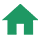JavaScript数据类型转换